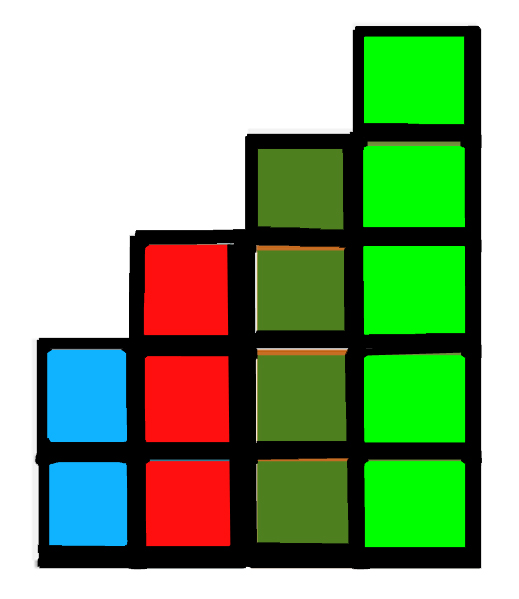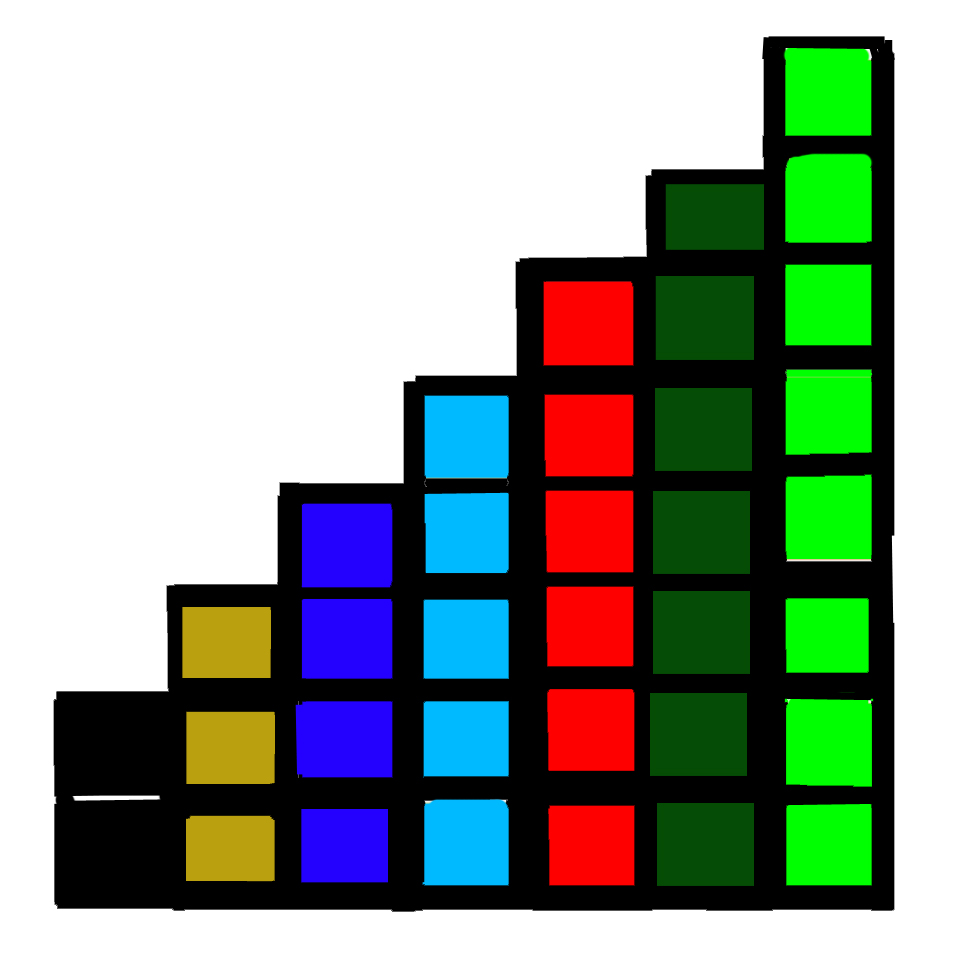Open in App
Not now

# Find the number of stair steps

• Difficulty Level : Medium
• Last Updated : 29 Sep, 2022

Given the total number of bricks T, find the number of stair steps that can be formed by using given bricks such that if step S has bricks B, then step S+1 should have exactly B+1 bricks and total number of bricks used should be less than or equal to number of bricks available.

Note: Number of bricks required to make step 1 of stair is 2, i.e step S = 1 must have exactly B = 2 bricks.

Examples:

Input: 15
Output: 4
Bricks should be arranged in this pattern to solve for T = 15:```Explanation:
Number of bricks at step increases by one.
At Step 1, Number of bricks = 2, Total = 2
At step 2, Number of bricks = 3, Total = 5
At step 3, Number of bricks = 4, Total = 9
At step 4, Number of bricks = 5, Total = 14

If we add 6 more bricks to form new step,
then the total number of bricks available will surpass.
Hence, number of steps that can be formed are 4 and
number of bricks used are 14 and we are left with
1 brick which is useless.

Input  : 40
Output : 7
Bricks should be arranged in this pattern to solve for T = 40:``````Explanation:
At Step 1, Number of bricks = 2, Total = 2
At step 2, Number of bricks = 3, Total = 5
At step 3, Number of bricks = 4, Total = 9
At step 4, Number of bricks = 5, Total = 14
At step 5, Number of bricks = 6, Total = 20
At step 6, Number of bricks = 7, Total = 27
At step 7, Number of bricks = 8, Total = 35

If we add 9 more bricks to form new step,
then the total number of bricks available will surpass.
Hence, number of steps that can be formed are 7 and
number of bricks used are 35 and we are left with
5 bricks which are useless.```

Approach:
We are interested in the number of steps and we know that each step Si uses exactly Bi number of bricks. We can represent this problem as an equation:
n * (n + 1) / 2 = T (For Natural number series starting from 1, 2, 3, 4, 5 …)
n * (n + 1) = 2 * T
n-1 will represent our final solution because our series in problem starts from 2, 3, 4, 5…
Now, we just have to solve this equation and for that we can exploit binary search to find the solution to this equation. Lower and Higher bounds of binary search are 1 and T.

Below is the implementation for the above approach:

## C++

 `// C++ program to find the number of steps``#include ``using` `namespace` `std;` `// Modified Binary search function``// to solve the equation``int` `solve(``int` `low, ``int` `high, ``int` `T)``{``    ``while` `(low <= high) {``        ``int` `mid = (low + high) / 2;` `        ``// if mid is solution to equation``        ``if` `((mid * (mid + 1)) == T)``            ``return` `mid;` `        ``// if our solution to equation``        ``// lies between mid and mid-1``        ``if` `(mid > 0 && (mid * (mid + 1)) > T &&``                        ``(mid * (mid - 1)) <= T)``            ``return` `mid - 1;` `        ``// if solution to equation is``        ``// greater than mid``        ``if` `((mid * (mid + 1)) > T)``            ``high = mid - 1;` `        ``// if solution to equation is less``        ``// than mid``        ``else``            ``low = mid + 1;``    ``}``    ``return` `-1;``}` `// driver function``int` `main()``{``    ``int` `T = 15;` `    ``// call binary search method to``    ``// solve for limits 1 to T``    ``int` `ans = solve(1, T, 2 * T);` `    ``// Because our pattern starts from 2, 3, 4, 5...``    ``// so, we subtract 1 from ans``    ``if` `(ans != -1)``        ``ans--;` `    ``cout << ``"Number of stair steps = "``         ``<< ans << endl;``    ``return` `0;``}`

## Java

 `// Java program to find the number of steps``import` `java.util.*;``import` `java.lang.*;` `public` `class` `GfG {` `    ``// Modified Binary search function``    ``// to solve the equation``    ``public` `static` `int` `solve(``int` `low, ``int` `high, ``int` `T)``    ``{``        ``while` `(low <= high) {``            ``int` `mid = (low + high) / ``2``;` `            ``// if mid is solution to equation``            ``if` `((mid * (mid + ``1``)) == T)``                ``return` `mid;` `            ``// if our solution to equation``            ``// lies between mid and mid-1``            ``if` `(mid > ``0` `&& (mid * (mid + ``1``)) > T &&``                           ``(mid * (mid - ``1``)) <= T)``                ``return` `mid - ``1``;` `            ``// if solution to equation is``            ``// greater than mid``            ``if` `((mid * (mid + ``1``)) > T)``                ``high = mid - ``1``;` `            ``// if solution to equation is less``            ``// than mid``            ``else``                ``low = mid + ``1``;``        ``}``        ``return` `-``1``;``    ``}` `    ``// driver function``    ``public` `static` `void` `main(String argc[])``    ``{``        ``int` `T = ``15``;` `        ``// call binary search method to``        ``// solve for limits 1 to T``        ``int` `ans = solve(``1``, T, ``2` `* T);` `        ``// Because our pattern starts from 2, 3, 4, 5...``        ``// so, we subtract 1 from ans``        ``if` `(ans != -``1``)``            ``ans--;` `        ``System.out.println(``"Number of stair steps = "` `+ ans);``    ``}``}` `/* This code is Contributed by Sagar Shukla */`

## Python3

 `# Python3 code to find the number of steps` `# Modified Binary search function``# to solve the equation``def` `solve( low, high, T ):``    ` `    ``while` `low <``=` `high:``        ``mid ``=` `int``((low ``+` `high) ``/` `2``)``        ` `        ``# if mid is solution to equation``        ``if` `(mid ``*` `(mid ``+` `1``)) ``=``=` `T:``            ``return` `mid``        ` `        ``# if our solution to equation``        ``# lies between mid and mid-1``        ``if` `(mid > ``0` `and` `(mid ``*` `(mid ``+` `1``)) > T``                ``and` `(mid ``*` `(mid ``-` `1``)) <``=` `T) :``            ``return` `mid ``-` `1``                    ` `        ``# if solution to equation is``        ``# greater than mid``        ``if` `(mid ``*` `(mid ``+` `1``)) > T:``            ``high ``=` `mid ``-` `1``;``            ` `        ``# if solution to equation is``        ``# less than mid``        ``else``:``            ``low ``=` `mid ``+` `1``    ``return` `-``1``    ` `# driver code``T ``=` `15` `# call binary search method to``# solve for limits 1 to T``ans ``=` `solve(``1``, T, ``2` `*` `T)` `# Because our pattern starts from 2, 3, 4, 5...``# so, we subtract 1 from ans``if` `ans !``=` `-``1``:``    ``ans``-``=` `1` `print``(``"Number of stair steps = "``, ans)` `# This code is contributed by "Sharad_Bhardwaj".`

## C#

 `// C# program to find the number of steps``using` `System;` `public` `class` `GfG {` `    ``// Modified Binary search function``    ``// to solve the equation``    ``public` `static` `int` `solve(``int` `low, ``int` `high, ``int` `T)``    ``{``        ``while` `(low <= high) {``            ``int` `mid = (low + high) / 2;` `            ``// if mid is solution to equation``            ``if` `((mid * (mid + 1)) == T)``                ``return` `mid;` `            ``// if our solution to equation``            ``// lies between mid and mid-1``            ``if` `(mid > 0 && (mid * (mid + 1)) > T &&``                           ``(mid * (mid - 1)) <= T)``                ``return` `mid - 1;` `            ``// if solution to equation is``            ``// greater than mid``            ``if` `((mid * (mid + 1)) > T)``                ``high = mid - 1;` `            ``// if solution to equation is less``            ``// than mid``            ``else``                ``low = mid + 1;``        ``}``        ``return` `-1;``    ``}` `    ``// Driver function``    ``public` `static` `void` `Main()``    ``{``        ``int` `T = 15;` `        ``// call binary search method to``        ``// solve for limits 1 to T``        ``int` `ans = solve(1, T, 2 * T);` `        ``// Because our pattern starts``        ``//from 2, 3, 4, 5...``        ``// so, we subtract 1 from ans``        ``if` `(ans != -1)``            ``ans--;``        ``Console.WriteLine(``"Number of stair steps = "` `+ ans);``    ``}``}` `/* This code is Contributed by vt_m */`

## PHP

 ` 0 && (``\$mid` `* (``\$mid` `+ 1)) > ``\$T` `&&``                        ``(``\$mid` `* (``\$mid` `- 1)) <= ``\$T` `)``            ``return` `\$mid` `- 1;` `        ``// if solution to equation is``        ``// greater than mid``        ``if` `((``\$mid` `* (``\$mid` `+ 1)) > ``\$T``)``            ``\$high` `= ``\$mid` `- 1;` `        ``// if solution to``        ``// equation is less``        ``// than mid``        ``else``            ``\$low` `= ``\$mid` `+ 1;``    ``}``    ``return` `-1;``}` `    ``// Driver Code``    ``\$T` `= 15;` `    ``// call binary search``    ``// method to solve``    ``// for limits 1 to T``    ``\$ans` `= solve(1, ``\$T``, 2 * ``\$T``);` `    ``// Because our pattern``    ``// starts from 2, 3, 4, 5...``    ``// so, we subtract 1 from ans``    ``if` `(``\$ans` `!= -1)``        ``\$ans``--;` `    ``echo` `"Number of stair steps = "``, ``\$ans``, ``"\n"``;` `// This code is contributed by aj_36``?>`

## Javascript

 ``

Output:

`Number of stair steps = 4`

Time complexity: O(logT) for given input T
Auxiliary space: O(1)